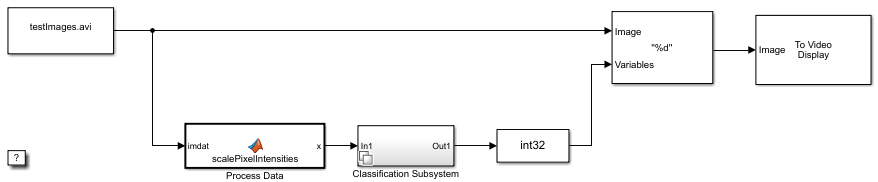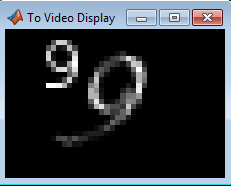# System Objects for Classification and Code Generation

This example shows how to generate C code from a MATLAB® System object™ that classifies images of digits by using a trained classification model. This example also shows how to use the System object for classification in Simulink®. The benefit of using System objects over MATLAB function is that System objects are more appropriate for processing large amounts of streaming data. For more details, see What Are System Objects?.

This example is based on Code Generation for Image Classification, which is an alternative workflow to Digit Classification Using HOG Features (Computer Vision Toolbox).

Load the `digitimages`.

`load digitimages.mat`

`images` is a 28-by-28-by-3000 array of `uint16` integers. Each page is a raster image of a digit. Each element is a pixel intensity. Corresponding labels are in the 3000-by-1 numeric vector `Y`. For more details, enter `Description` at the command line.

Store the number of observations and the number of predictor variables. Create a data partition that specifies to hold out 20% of the data. Extract training and test set indices from the data partition.

```rng(1); % For reproducibility n = size(images,3); p = numel(images(:,:,1)); cvp = cvpartition(n,'Holdout',0.20); idxTrn = training(cvp); idxTest = test(cvp);```

### Rescale Data

Rescale the pixel intensities so that they range in the interval [0,1] within each image. Specifically, suppose ${p}_{ij}$ is pixel intensity $j$ within image $i$. For image $i$, rescale all of its pixel intensities by using this formula:

`${\underset{}{\overset{ˆ}{p}}}_{ij}=\frac{{p}_{ij}-\underset{j}{\mathrm{min}}\left({p}_{ij}\right)}{\underset{j}{\mathrm{max}}\left({p}_{ij}\right)-\underset{j}{\mathrm{min}}\left({p}_{ij}\right)}.$`

```X = double(images); for i = 1:n minX = min(min(X(:,:,i))); maxX = max(max(X(:,:,i))); X(:,:,i) = (X(:,:,i) - minX)/(maxX - minX); end```

### Reshape Data

For code generation, the predictor data for training must be in a table of numeric variables or a numeric matrix.

Reshape the data to a matrix such that predictor variables correspond to columns and images correspond to rows. Because `reshape` takes elements column-wise, transpose its result.

`X = reshape(X,[p,n])';`

### Train and Optimize Classification Models

Cross-validate an ECOC model of SVM binary learners and a random forest based on the training observations. Use 5-fold cross-validation.

For the ECOC model, specify predictor standardization and optimize classification error over the ECOC coding design and the SVM box constraint. Explore all combinations of these values:

• For the ECOC coding design, use one-versus-one and one-versus-all.

• For the SVM box constraint, use three logarithmically spaced values from 0.1 to 100 each. For all models, store the 5-fold cross-validated misclassification rates.

```coding = {'onevsone' 'onevsall'}; boxconstraint = logspace(-1,2,3); cvLossECOC = nan(numel(coding),numel(boxconstraint)); % For preallocation for i = 1:numel(coding) for j = 1:numel(boxconstraint) t = templateSVM('BoxConstraint',boxconstraint(j),'Standardize',true); CVMdl = fitcecoc(X(idxTrn,:),Y(idxTrn),'Learners',t,'KFold',5,... 'Coding',coding{i}); cvLossECOC(i,j) = kfoldLoss(CVMdl); fprintf('cvLossECOC = %f for model using %s coding and box constraint=%f\n',... cvLossECOC(i,j),coding{i},boxconstraint(j)) end end```
```cvLossECOC = 0.058333 for model using onevsone coding and box constraint=0.100000 cvLossECOC = 0.057083 for model using onevsone coding and box constraint=3.162278 cvLossECOC = 0.050000 for model using onevsone coding and box constraint=100.000000 cvLossECOC = 0.120417 for model using onevsall coding and box constraint=0.100000 cvLossECOC = 0.121667 for model using onevsall coding and box constraint=3.162278 cvLossECOC = 0.127917 for model using onevsall coding and box constraint=100.000000 ```

For the random forest, vary the maximum number of splits by using the values in the sequence $\left\{{3}^{2},{3}^{3},...,{3}^{m}\right\}$. m is such that ${3}^{m}$ is no greater than n - 1. To reproduce random predictor selections, specify `'Reproducible',true`.

```n = size(X,1); m = floor(log(n - 1)/log(3)); maxNumSplits = 3.^(2:m); cvLossRF = nan(numel(maxNumSplits)); for i = 1:numel(maxNumSplits) t = templateTree('MaxNumSplits',maxNumSplits(i),'Reproducible',true); CVMdl = fitcensemble(X(idxTrn,:),Y(idxTrn),'Method','bag','Learners',t,... 'KFold',5); cvLossRF(i) = kfoldLoss(CVMdl); fprintf('cvLossRF = %f for model using %d as the maximum number of splits\n',... cvLossRF(i),maxNumSplits(i)) end```
```cvLossRF = 0.319167 for model using 9 as the maximum number of splits cvLossRF = 0.192917 for model using 27 as the maximum number of splits cvLossRF = 0.066250 for model using 81 as the maximum number of splits cvLossRF = 0.015000 for model using 243 as the maximum number of splits cvLossRF = 0.013333 for model using 729 as the maximum number of splits cvLossRF = 0.009583 for model using 2187 as the maximum number of splits ```

For each algorithm, determine the hyperparameter indices that yield the minimal misclassification rates.

`minCVLossECOC = min(cvLossECOC(:))`
```minCVLossECOC = 0.0500 ```
```linIdx = find(cvLossECOC == minCVLossECOC,1); [bestI,bestJ] = ind2sub(size(cvLossECOC),linIdx); bestCoding = coding{bestI}```
```bestCoding = 'onevsone' ```
`bestBoxConstraint = boxconstraint(bestJ)`
```bestBoxConstraint = 100 ```
`minCVLossRF = min(cvLossRF(:))`
```minCVLossRF = 0.0096 ```
```linIdx = find(cvLossRF == minCVLossRF,1); [bestI,bestJ] = ind2sub(size(cvLossRF),linIdx); bestMNS = maxNumSplits(bestI)```
```bestMNS = 2187 ```

The random forest achieves a smaller cross-validated misclassification rate.

Train an ECOC model and a random forest using the training data. Supply the optimal hyperparameter combinations.

```t = templateSVM('BoxConstraint',bestBoxConstraint,'Standardize',true); MdlECOC = fitcecoc(X(idxTrn,:),Y(idxTrn),'Learners',t,'Coding',bestCoding); t = templateTree('MaxNumSplits',bestMNS); MdlRF = fitcensemble(X(idxTrn,:),Y(idxTrn),'Method','bag','Learners',t);```

Create a variable for the test sample images and use the trained models to predict test sample labels.

```testImages = X(idxTest,:); testLabelsECOC = predict(MdlECOC,testImages); testLabelsRF = predict(MdlRF,testImages);```

### Save Classification Model to Disk

`MdlECOC` and `MdlRF` are predictive classification models, but you must prepare them for code generation. Save `MdlECOC` and `MdlRF` to your present working folder using `saveLearnerForCoder`.

```saveLearnerForCoder(MdlECOC,'DigitImagesECOC'); saveLearnerForCoder(MdlRF,'DigitImagesRF');```

### Create System Object for Prediction

Create two System objects, one for the ECOC model and the other for the random forest, that:

• Load the previously saved trained model by using `loadLearnerForCoder`.

• Make sequential predictions by the `step` method.

• Enforce no size changes to the input data.

• Enforce double-precision, scalar output.

`type ECOCClassifier.m % Display contents of ECOCClassifier.m file`
```classdef ECOCClassifier < matlab.System % ECOCCLASSIFIER Predict image labels from trained ECOC model % % ECOCCLASSIFIER loads the trained ECOC model from % |'DigitImagesECOC.mat'|, and predicts labels for new observations % based on the trained model. The ECOC model in % |'DigitImagesECOC.mat'| was cross-validated using the training data % in the sample data |digitimages.mat|. properties(Access = private) CompactMdl % The compacted, trained ECOC model end methods(Access = protected) function setupImpl(obj) % Load ECOC model from file obj.CompactMdl = loadLearnerForCoder('DigitImagesECOC'); end function y = stepImpl(obj,u) y = predict(obj.CompactMdl,u); end function flag = isInputSizeMutableImpl(obj,index) % Return false if input size is not allowed to change while % system is running flag = false; end function dataout = getOutputDataTypeImpl(~) dataout = 'double'; end function sizeout = getOutputSizeImpl(~) sizeout = [1 1]; end end end ```
`type RFClassifier.m % Display contents of RFClassifier.m file`
```classdef RFClassifier < matlab.System % RFCLASSIFIER Predict image labels from trained random forest % % RFCLASSIFIER loads the trained random forest from % |'DigitImagesRF.mat'|, and predicts labels for new observations based % on the trained model. The random forest in |'DigitImagesRF.mat'| % was cross-validated using the training data in the sample data % |digitimages.mat|. properties(Access = private) CompactMdl % The compacted, trained random forest end methods(Access = protected) function setupImpl(obj) % Load random forest from file obj.CompactMdl = loadLearnerForCoder('DigitImagesRF'); end function y = stepImpl(obj,u) y = predict(obj.CompactMdl,u); end function flag = isInputSizeMutableImpl(obj,index) % Return false if input size is not allowed to change while % system is running flag = false; end function dataout = getOutputDataTypeImpl(~) dataout = 'double'; end function sizeout = getOutputSizeImpl(~) sizeout = [1 1]; end end end ```

Note: If you click the button located in the upper-right section of this page and open this example in MATLAB®, then MATLAB® opens the example folder. This folder includes the files used in this example.

For System object basic requirements, see Define Basic System Objects.

### Define Prediction Functions for Code Generation

Define two MATLAB functions called `predictDigitECOCSO.m` and `predictDigitRFSO.m`. The functions:

• Include the code generation directive `%#codegen`.

• Accept image data commensurate with `X`.

• Predict labels using the `ECOCClassifier` and `RFClassifier` System objects, respectively.

• Return predicted labels.

`type predictDigitECOCSO.m % Display contents of predictDigitECOCSO.m file`
```function label = predictDigitECOCSO(X) %#codegen %PREDICTDIGITECOCSO Classify digit in image using ECOC Model System object % PREDICTDIGITECOCSO classifies the 28-by-28 images in the rows of X % using the compact ECOC model in the System object ECOCClassifier, and % then returns class labels in label. classifier = ECOCClassifier; label = step(classifier,X); end ```
`type predictDigitRFSO.m % Display contents of predictDigitRFSO.m file`
```function label = predictDigitRFSO(X) %#codegen %PREDICTDIGITRFSO Classify digit in image using RF Model System object % PREDICTDIGITRFSO classifies the 28-by-28 images in the rows of X % using the compact random forest in the System object RFClassifier, and % then returns class labels in label. classifier = RFClassifier; label = step(classifier,X); end ```

### Compile MATLAB Function to MEX File

Compile the prediction function that achieves better test-sample accuracy to a MEX file by using `codegen`. Specify the test set images by using the `-args` argument.

```if(minCVLossECOC <= minCVLossRF) codegen predictDigitECOCSO -args testImages else codegen predictDigitRFSO -args testImages end```
```Code generation successful. ```

Verify that the generated MEX file produces the same predictions as the MATLAB function.

```if(minCVLossECOC <= minCVLossRF) mexLabels = predictDigitECOCSO_mex(testImages); verifyMEX = sum(mexLabels == testLabelsECOC) == numel(testLabelsECOC) else mexLabels = predictDigitRFSO_mex(testImages); verifyMEX = sum(mexLabels == testLabelsRF) == numel(testLabelsRF) end```
```verifyMEX = logical 1 ```

`verifyMEX` is `1`, which indicates that the predictions made by the generated MEX file and the corresponding MATLAB function are the same.

### Predict Labels by Using System Objects in Simulink

Create a video file that displays the test-set images frame-by-frame.

```v = VideoWriter('testImages.avi','Uncompressed AVI'); v.FrameRate = 1; open(v); dim = sqrt(p)*[1 1]; for j = 1:size(testImages,1) writeVideo(v,reshape(testImages(j,:),dim)); end close(v);```

Define a function called `scalePixelIntensities.m` that converts RGB images to grayscale, and then scales the resulting pixel intensities so that their values are in the interval [0,1].

`type scalePixelIntensities.m % Display contents of scalePixelIntensities.m file`
```function x = scalePixelIntensities(imdat) %SCALEPIXELINTENSITIES Scales image pixel intensities % SCALEPIXELINTENSITIES scales the pixel intensities of the image such % that the result x is a row vector of values in the interval [0,1]. imdat = rgb2gray(imdat); minimdat = min(min(imdat)); maximdat = max(max(imdat)); x = (imdat - minimdat)/(maximdat - minimdat); end ```

Load the Simulink® model `slexClassifyAndDisplayDigitImages.slx`.

```SimMdlName = 'slexClassifyAndDisplayDigitImages'; open_system(SimMdlName);```The figure displays the Simulink® model. At the beginning of simulation, the From Multimedia File block loads the video file of the test-set images. For each image in the video:

• The From Multimedia File block converts and outputs the image to a 28-by-28 matrix of pixel intensities.

• The Process Data block scales the pixel intensities by using `scalePixelIntensities.m`, and outputs a 1-by-784 vector of scaled intensities.

• The Classification Subsystem block predicts labels given the processed image data. The block chooses the System object that minimizes classification error. In this case, the block chooses the random forest. The block outputs a double-precision scalar label.

• The Data Type Conversion block converts the label to an `int32` scalar.

• The Insert Text block embeds the predicted label on the current frame.

• The To Video Display block displays the annotated frame.

Simulate the model.

`sim(SimMdlName)`The model displays all 600 test-set images and its prediction quickly. The last image remains in the video display. You can generate predictions and display them with corresponding images one-by-one by clicking the Step Forward button instead.

If you also have a Simulink® Coder™ license, then you can generate C code from `slexClassifyAndDisplayDigitImages.slx` in Simulink® or from the command line using `slbuild` (Simulink). For more details, see Generate C Code for a Model (Simulink Coder).